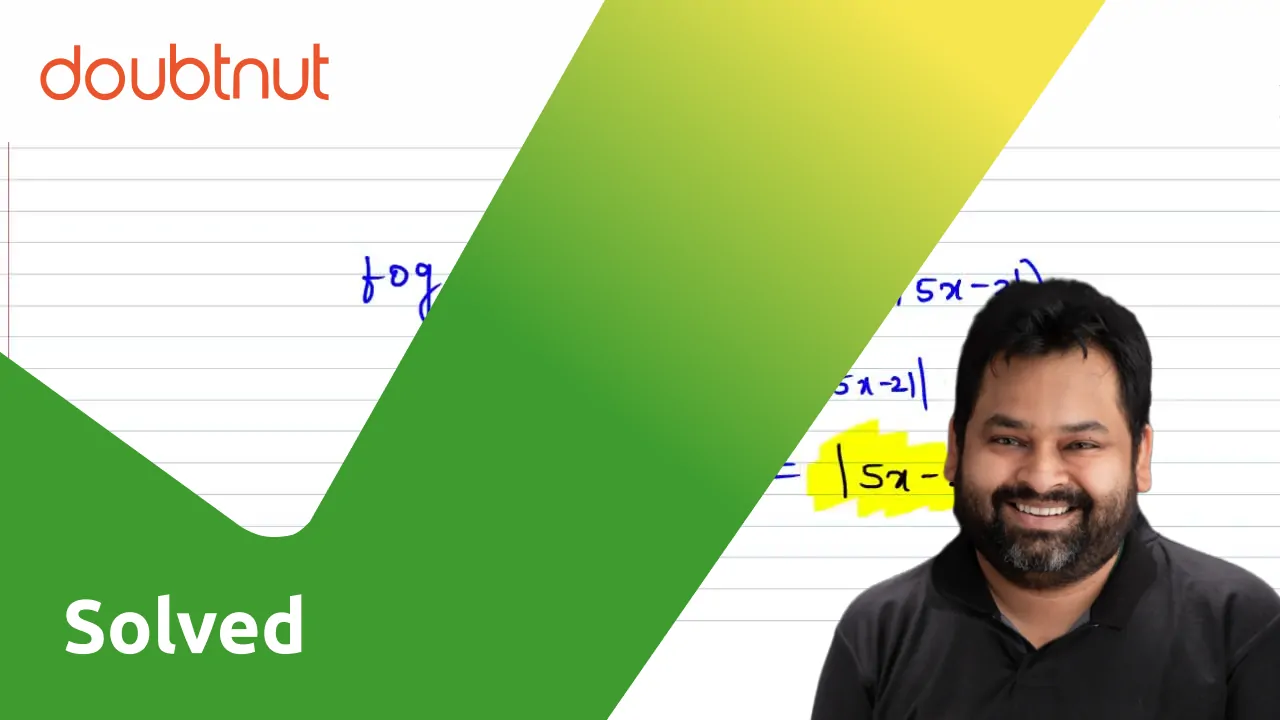Doubtnut App - Get 50 Lakh+ video solutions better than GoogleInstall Now

# Find gof and fog , if f:R→R and g:R→R are given by f(x)=|x| and g(x)=|5x−2| .

Text Solution
Verified by Experts

## Given: f(x)=|x| and g(x)=|5x−2| f(g(x))=|g(x)| fog(x)=||5x−2|| fog(x)=|5x−2| g(f(x))=|5f(x)−2| gof(x)=|5|x|−2|